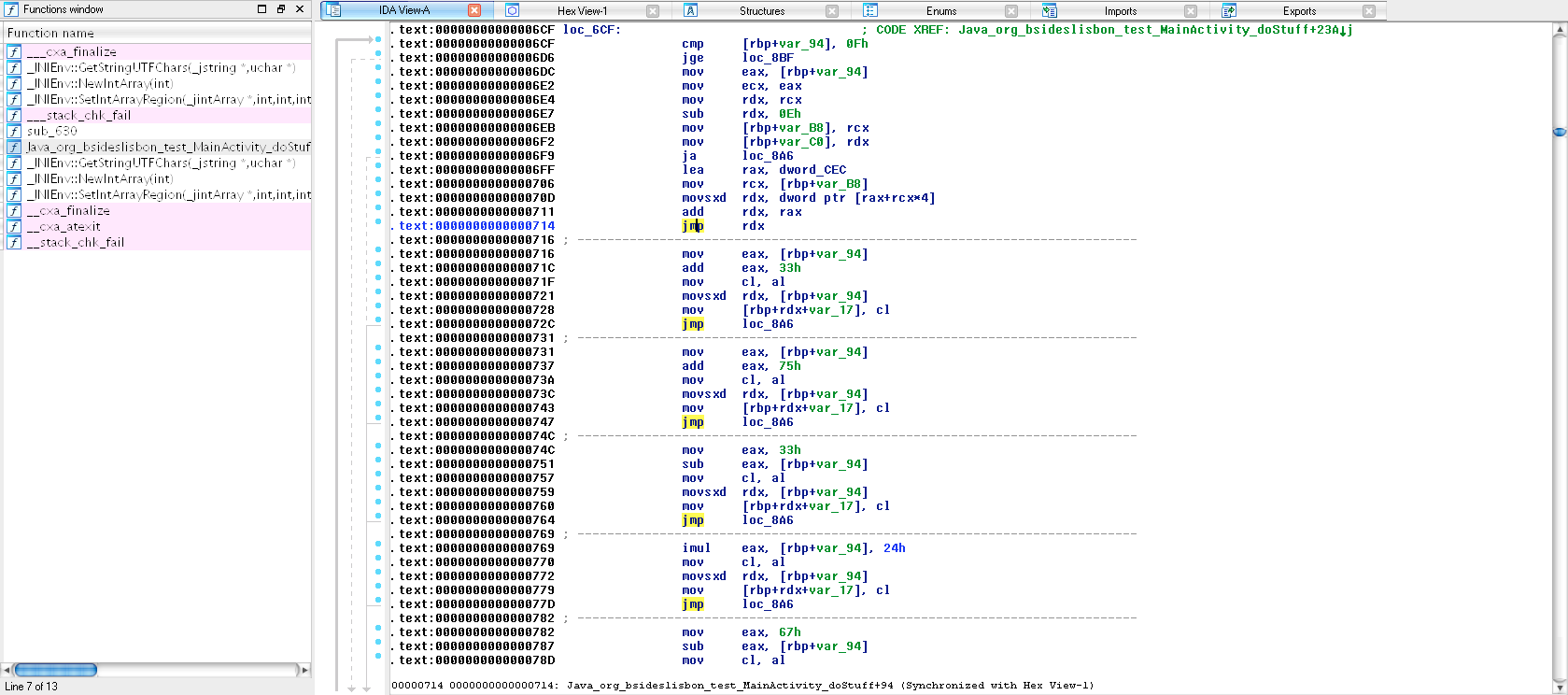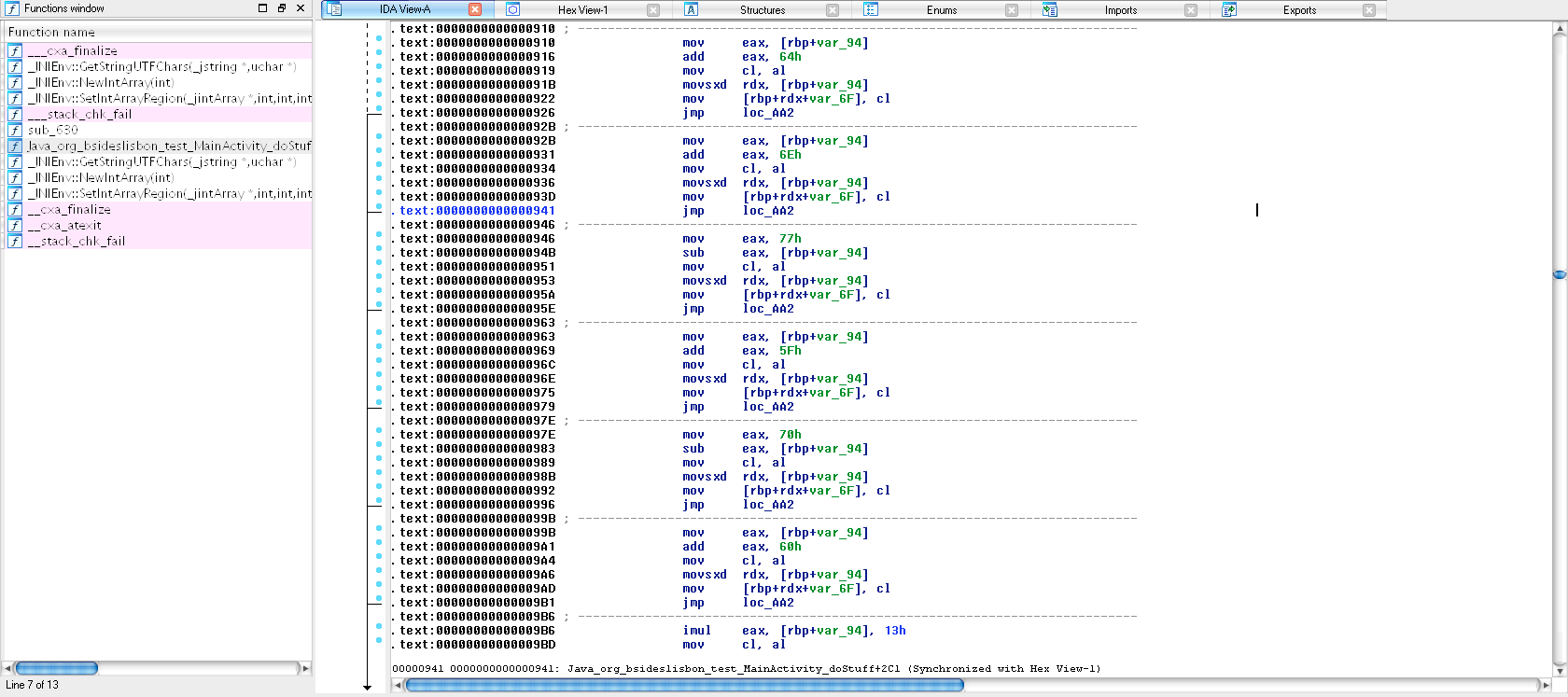# BSides Lisbon CTF 2016 Writeup

## E-Cryption - Reverse 400

Posted by 0xacb on November 12, 2016 · 6 mins read

Yesterday, I went to BSides Lisbon and my team finished the CTF in second place! Thanks to all the organizers for putting so much effort in the best infosec event of the year in Portugal.

The E-Cryption challenge was baked by Cláudio André @clviper and it was one of my favorites. I solved this statically, i.e., without running/debugging the app. Let's see how to solve it.

We were given two files: `e-corp.apk` and `e-crypted message`. The e-crypted message was just a text file containing the string: "2f2936516c2779303c35446c693b75". So, our goal is to decrypt the ciphertext, 30 hexadecimals long, probably not a result of a hash function.

I started by decompiling the APK using the JADX decompiler. We can realize what the app does inside the package `org.bsideslisbon.test`. There are some interesting functions in `MainActivity.java`:

``````public void onClick(View v) {
String hexed = BuildConfig.FLAVOR;
int[] output = MainActivity.this.doStuff(this.val\$input.getText().toString());
for (int i = 0; i < 15; i++) {
hexed = hexed + String.format("%02X", new Object[]{Integer.valueOf(output[i] & MotionEventCompat.ACTION_MASK)});
}
this.val\$tv.setText(hexed);
}
``````

This function basically calls `doStuff` with the input string as argument. Then, `doStuff` returns an array of 15 integers, which is hex encoded and displayed. However there is no decompiled java code for `doStuff`.

``````public native int[] doStuff(String str);

...

static {
}
``````

Damn, it's a native function! We need to reverse a native library. To get the library you just need to unzip the APK file. In the lib directory, we have more directories: arm64-v8a, armeabi, armeabi-v7a, mips, mips64, x86 and x86_64. Each one of them contains a `libnative-lib.so` for the corresponding architecture. I decided to reverse x86_64 since I'm used to x86_64 assembly.

``````_DWORD dword_CB0 =
{
4294966368,
4294966395,
4294966422,
4294966451,
4294966478,
4294966507,
4294966534,
4294966559,
4294966586,
4294966613,
4294966640,
4294966667,
4294966694,
4294966721,
4294966748
};
_DWORD dword_CEC =
{
4294965802,
4294965829,
4294965856,
4294965885,
4294965910,
4294965939,
4294965966,
4294965991,
4294966018,
4294966045,
4294966072,
4294966099,
4294966126,
4294966153,
4294966180
};

...

__int64 __fastcall Java_org_bsideslisbon_test_MainActivity_doStuff(_JNIEnv *a1, __int64 a2, __int64 a3)
{
__int64 v3;
__int64 result;
__int64 v5;
signed int i;
signed int j;
signed int k;
signed int l;
__int64 v10;
char v11;
int v12;
char v13;
__int64 v14;

v14 = *MK_FP(__FS__, 40LL);

LODWORD(v3) = _JNIEnv::GetStringUTFChars(a1, a3, 0LL);
v10 = v3;
for ( i = 0; i < 15; ++i )
{
if ( (unsigned int)i <= 0xEuLL )
JUMPOUT(__CS__, (char *)dword_CEC + dword_CEC[(unsigned __int64)(unsigned int)i]);
}

for ( j = 0; j < 15; ++j )
{
if ( (unsigned int)j <= 0xEuLL )
JUMPOUT(__CS__, (char *)dword_CB0 + dword_CB0[(unsigned __int64)(unsigned int)j]);
}

v5 = _JNIEnv::NewIntArray(a1, 60);

for ( k = 0; k <= 14; ++k )
v12[k] = *(_BYTE *)(v10 + k) ^ v13[k];
for ( l = 0; l <= 14; ++l )
v12[l] ^= v11[l];

_JNIEnv::SetIntArrayRegion(a1, v5, 0LL, 60LL, v12);

result = v5;
if ( *MK_FP(__FS__, 40LL) == v14 )
result = v5;
return result;
}
``````

First, it calls `_JNIEnv::GetStringUTFChars` and stores the result in `v10`, which is a pointer to a char array, since the next operation involving `v10` is `*(_BYTE *)(v10 + k)`. So, `v10` is our input as a char array. Then, we have two weird for loops with some jumps: IDA couldn't decompile them (100%) correctly. Let's leave these two for loops in stand by and keep going.

Now we have two more loops, the iterations of the first one can be translated as `v12[k] = v10[k] ^ v13[k]` and the second loop it's just the same as `v12[l] = v12[l] ^ v11[l]`. The result of these operations, the ciphertext, is stored in `v12`. However, we don't have the values of `v13` and `v11`. Maybe the first loops are responsible for the construction of these arrays in runtime. Let's hope that they don't change according to the input, that would be evil 😈

In order to find the values of `v11` and `v13` I analyzed assembly in IDA PRO and realized what those jumps were doing: constructing `v11` and `v13`!In the first loop, `rbp+var_94` is the `i` variable and `rbp+rdx+var_17` points to `v11[i]`. In the second loop, `rbp+rdx+var_6F` points to `v13[i]`. I translated all these instructions to python, and ended up with my final solution:

``````v11 = [0x33, 0x75+1, 0x33-2, 0x24*3, 0x67-4, 0x2b+5, 0x13*6, 0x69+7, 0x28+8, 0x6e+9, 0x64+10, 0x68+11, 0x6d+12, 0x23+13, 0x67+14]
v13 = [0x64, 0x6e+1, 0x77-2, 0x5f+3, 0x70-4, 0x60+5, 0x13*6, 0x29+7, 0x70+8, 0x69+9, 0x6b+10, 0x61+11, 0x59+12, 0x66+13, 0x13+14]

def encrypt(s):
v12 = *15
v10 = list(s)
for k in range(0, 15):
v12[k] = ord(v10[k]) ^ v13[k]
for l in range(0, 15):
v12[l] = v12[l] ^ v11[l]
return "".join(map(chr, v12)).encode("hex")

def decrypt(d):
v10 = *15
v12 = list(d.decode("hex"))
for l in range(0, 15):
v12[l] = ord(v12[l]) ^ v11[l]
for k in range(0, 15):
v10[k] = v12[k] ^ v13[k]
return "".join(map(chr, v10))

if __name__ == "__main__":
assert (decrypt(encrypt("bsideslisbonctf")) == "bsideslisbonctf")

print("Flag: %s" % decrypt("2f2936516c2779303c35446c693b75"))``````
```Flag: x0r_crypt0_sux!
```

Gotcha! 🙂